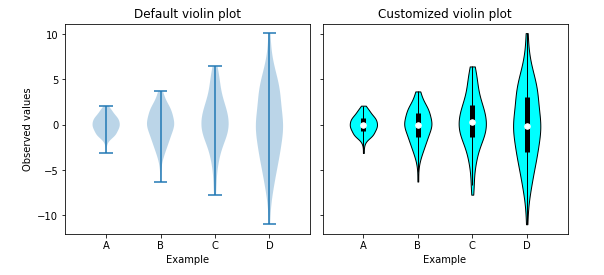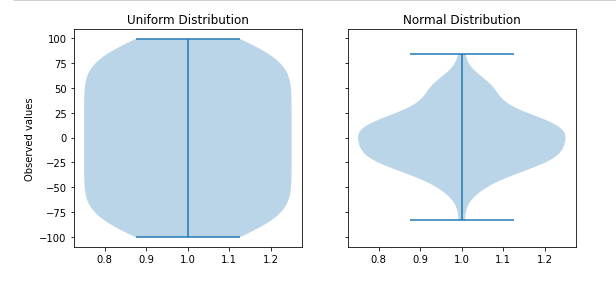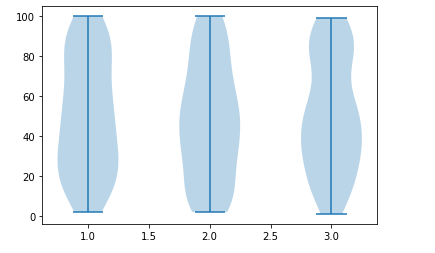# Introduction

In this article, we will go through the tutorial for the matplotlib violin plot for your data science and machine learning projects. We will talk about the varieties of violin charts that can be used in various ways. Throughout this matplotlib tutorial of violin charts, you’ll learn about the details that should be taken care of while working with such an interesting feature of the matplotlib library.

### Importing Matplotlib Library

Before beginning with this matplotlib bar plot tutorial, we’ll need the matplotlib and other libraries.

In :

```import numpy as np
import matplotlib.pyplot as plt```

### Matplotlib Violin Plot Syntax

Axes.violinplot(self, dataset, positions=None, vert=True, widths=0.5, showmeans=False, showextrema=True, showmedians=False, quantiles=None, points=100, bw_method=None, *, data=None)

• dataset : Array or sequence of vector – The Input data is provided from here.
• positions : Array-like – It sets the default positions of violin plots.
• vert : bool – If true, creates a vertical violin plot. Otherwise, creates a horizontal violin plot.
• widths : Array-like – A scalar or a vector that sets the maximal width of each violin.
• showmeans : bool, default : False – If True, will render means data
• showextrema : bool, default : True – Helps in rendering extreme values
• showmedians : : bool, default : False – It will render medians
• quantiles : Array-like, default : None – Used for setting quantiles in violin plots

### Example 1: Simple Matplotlib Violin Plot based on Dataset

The two examples shown are the simple violin plots where the one on the left is without any customizations whereas the other one has customizations like change in color, the information mean, median, and outliers is also conveyed.

The violinplot helps in plotting these violin plots. We use some custom build functions for setting the axis style and adjacent values.

With the help of the dataset generated, we can easily plot the violin plots. We can also display the mean, median information along with these violin plots.

In :
```import matplotlib.pyplot as plt
import numpy as np

upper_adjacent_value = q3 + (q3 - q1) * 1.5

lower_adjacent_value = q1 - (q3 - q1) * 1.5

def set_axis_style(ax, labels):
ax.get_xaxis().set_tick_params(direction='out')
ax.xaxis.set_ticks_position('bottom')
ax.set_xticks(np.arange(1, len(labels) + 1))
ax.set_xticklabels(labels)
ax.set_xlim(0.25, len(labels) + 0.75)
ax.set_xlabel('Example')

# create test data
np.random.seed(19680801)
data = [sorted(np.random.normal(0, std, 100)) for std in range(1, 5)]

fig, (ax1, ax2) = plt.subplots(nrows=1, ncols=2, figsize=(9, 4), sharey=True)

ax1.set_title('Default violin plot')
ax1.set_ylabel('Observed values')
ax1.violinplot(data)

ax2.set_title('Customized violin plot')
parts = ax2.violinplot(
data, showmeans=False, showmedians=False,
showextrema=False)

for pc in parts['bodies']:
pc.set_facecolor('Cyan')
pc.set_edgecolor('black')
pc.set_alpha(1)

quartile1, medians, quartile3 = np.percentile(data, [25, 50, 75], axis=1)
whiskers = np.array([
for sorted_array, q1, q3 in zip(data, quartile1, quartile3)])
whiskers_min, whiskers_max = whiskers[:, 0], whiskers[:, 1]

inds = np.arange(1, len(medians) + 1)
ax2.scatter(inds, medians, marker='o', color='white', s=30, zorder=3)
ax2.vlines(inds, quartile1, quartile3, color='k', linestyle='-', lw=5)
ax2.vlines(inds, whiskers_min, whiskers_max, color='k', linestyle='-', lw=1)

# set style for the axes
labels = ['A', 'B', 'C', 'D']
for ax in [ax1, ax2]:
set_axis_style(ax, labels)

plt.show()
```
Output:### Example 2: Matplotlib Violin Plot Showing Types of Distribution

Violin Plots are helpful in showing the type of distribution a particular dataset possesses. We first generate uniformly distributed and normally distributed data.

Then we call the violinplot function to pass these two different types of datasets.

In :
```# creating a list of
# uniformly distributed values
uniform = np.arange(-100, 100)

# creating a list of normally
# distributed values
normal = np.random.normal(size = 100)*30

# creating figure and axes to
# plot the image
fig, (ax1, ax2) = plt.subplots(nrows = 1, ncols = 2, figsize =(9, 4), sharey = True)

# plotting violin plot for
# uniform distribution
ax1.set_title('Uniform Distribution')
ax1.set_ylabel('Observed values')
ax1.violinplot(uniform)

# plotting violin plot for
# normal distribution
ax2.set_title('Normal Distribution')
ax2.violinplot(normal)

# Function to show the plot
plt.show()
```
Output:### Example 3: Matplotlib Violin Plot of Varying Sizes

This last example of the violin plot tutorial will showcase how one can build violin plots with varying sizes. For this again data is generated using random function.

The data is provided to the violinplot function in the form of lists.

In :
```from random import randint

# Creating 3 empty lists
l1 = []
l2 =[]
l3 =[]

# Filling the lists with random value
for i in range(100):
n = randint(1, 100)
l1.append(n)

for i in range(100):
n = randint(1, 100)
l2.append(n)

for i in range(100):
n = randint(1, 100)
l3.append(n)

random_collection = [l1, l2, l3]

# Create a figure instance
fig = plt.figure()

# Create an axes instance
ax = fig.gca()

# Create the violinplot
violinplot = ax.violinplot(random_collection)
plt.show()
```
Output:## Conclusion

We have reached the end of this tutorial of the matplotlib violin plot where we saw different types of examples. We talked about the intricate details that should be taken care of while building a variety of useful violin plots. We also looked at how we can play around with the parameters for producing a combination of different surface plots using matplotlib.

Reference –  Matplotlib Documentation